# Equation

Equation

In this mini-lesson, we will explore the concept of equations by knowing the definition of an equation, equation examples, equation of a circle, quadratic equation, linear equation, and types of equations.

Before we get started, let's see what Joe did.

Joe created a game called 'Mind Reader.'

He wants to play it with his friends.

He asks one of his friends, Kaira, to think of a number, multiply it by 2, and subtract 5 from it.

He asks her the final result.

Kaira says, 'It is 13.'Joe instantly says that the number Kaira thought of initially was 9

Kaira nods and Joe's friends including Kaira are surprised!

Everybody wants to know how the game 'Mind Reader' works.

Do you know how it works?

By the end of this short lesson, you will understand how it works.

## Lesson Plan

 1 What are Equations? 2 Solved Examples on Equations 3 Interactive Questions on Equations

## What are Equations?

### Meaning of Equation in Maths

An equation is a mathematical statement with an 'equal to' symbol between two algebraic expressions that have equal values.

For example, $$2x+9$$ is the expression on the left-hand side that is equated with the expression 24 on the right-hand side.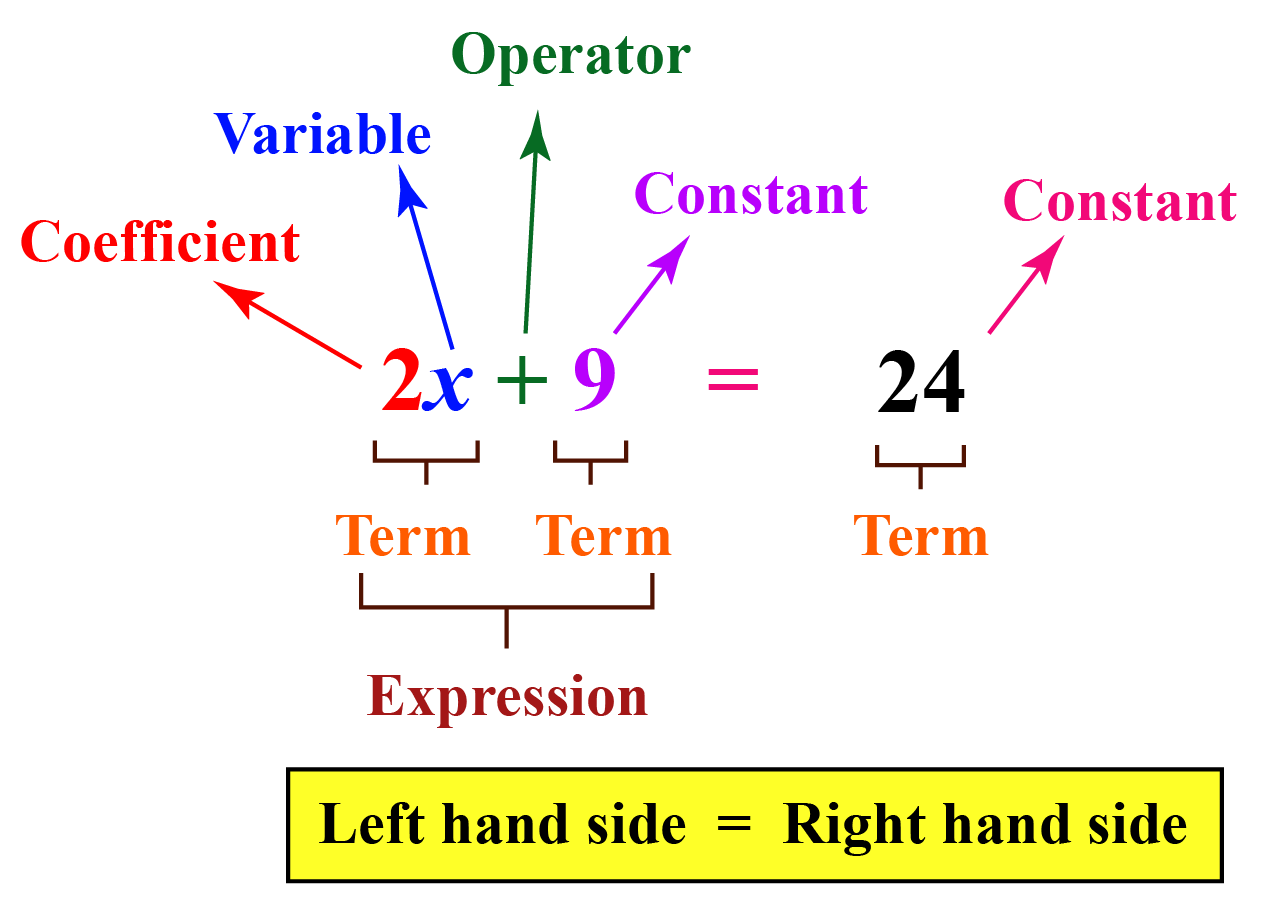Look at the following examples. These will give you an idea of the meaning of an equation in math.

Equations Is it an equation?
1. $$y=8x-9$$ Yes
2. $$y+x^2-7$$ No, because there is no 'equal to' symbol.
3. $$7+2=10-1$$ Yes

## How Do You Solve Equations?

An equation is like a weighing balance with equal weights on both sides.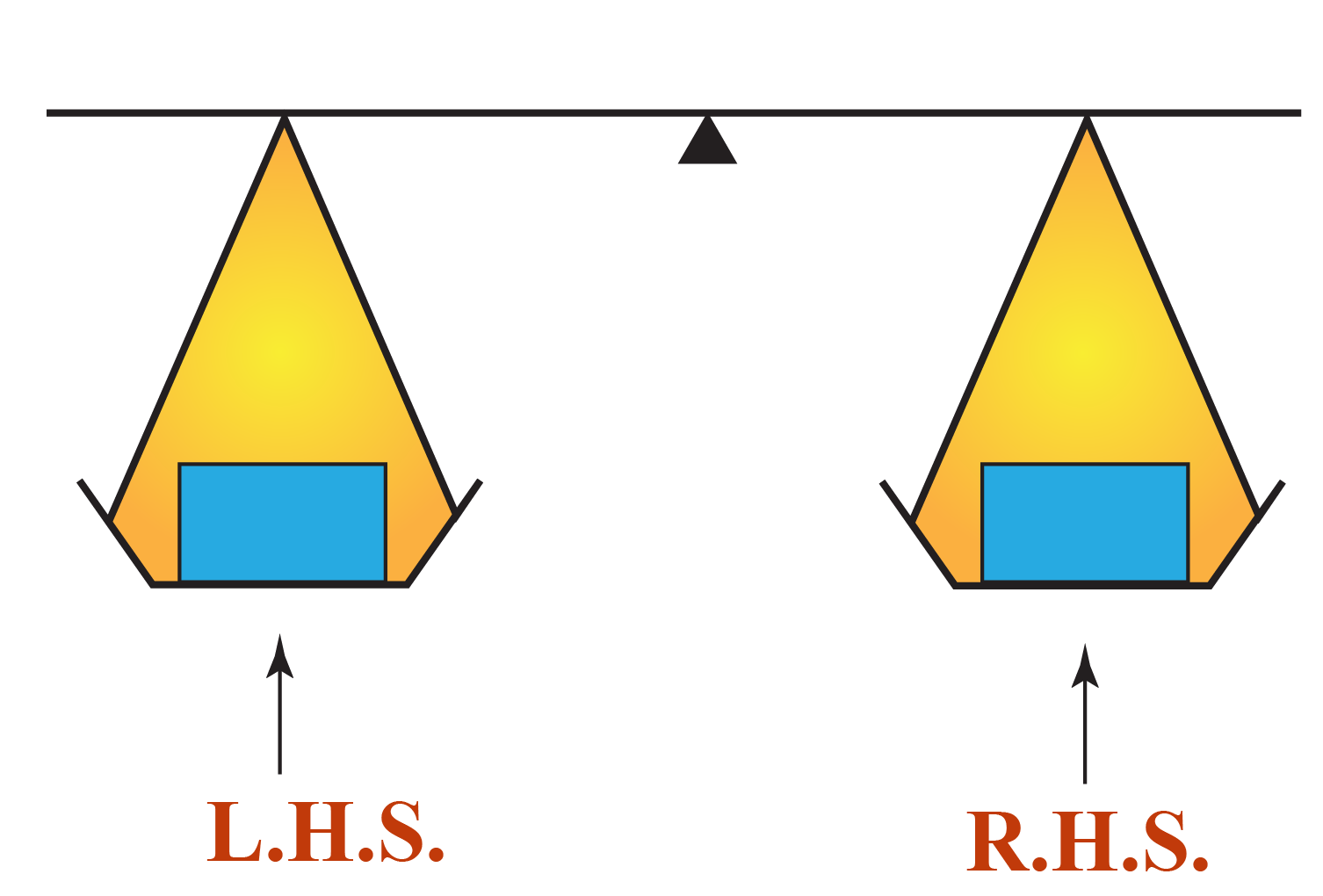If we add or subtract the same number from both sides of an equation, it still holds.

Similarly, if we multiply or divide the same number on both sides of an equation, it still holds.

Consider the equation of a line, $$3x-2=4$$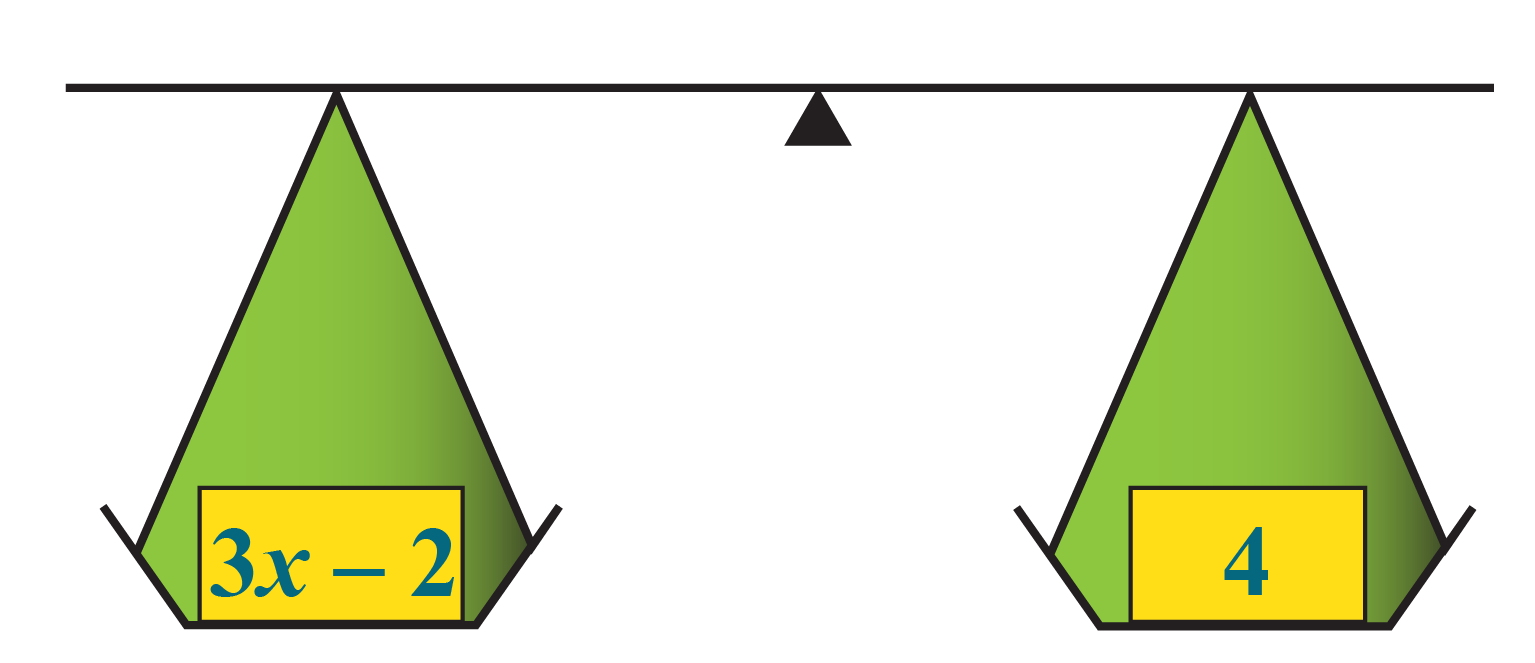We will perform mathematical operations on the LHS and the RHS so that the balance is not disturbed.

Let's add 2 on both sides to reduce the LHS to $$3x$$

This will not disturb the balance.

The new LHS is $$3x-2+2=3x$$ and the new RHS is $$4+2=6$$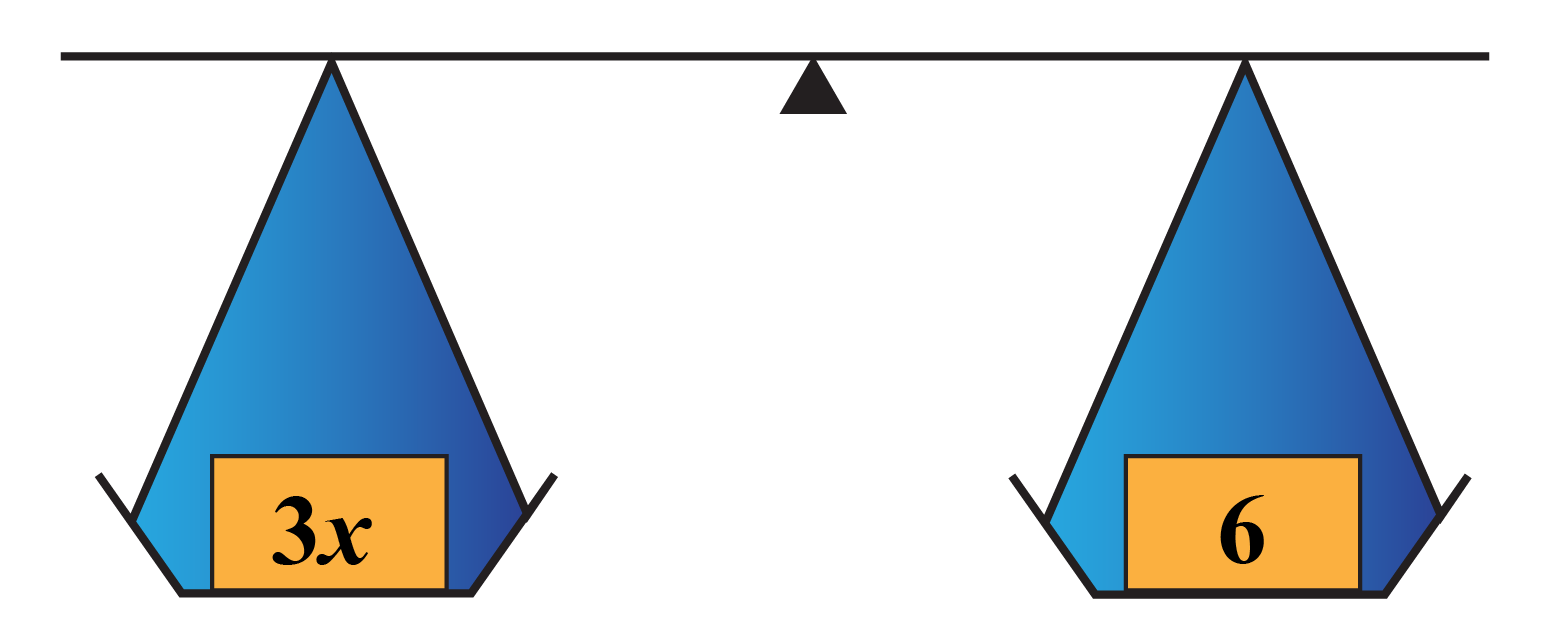Now, let's divide both sides by 3 to reduce the LHS to $$x$$

Thus, the solution of the equation of a line is $$x=2$$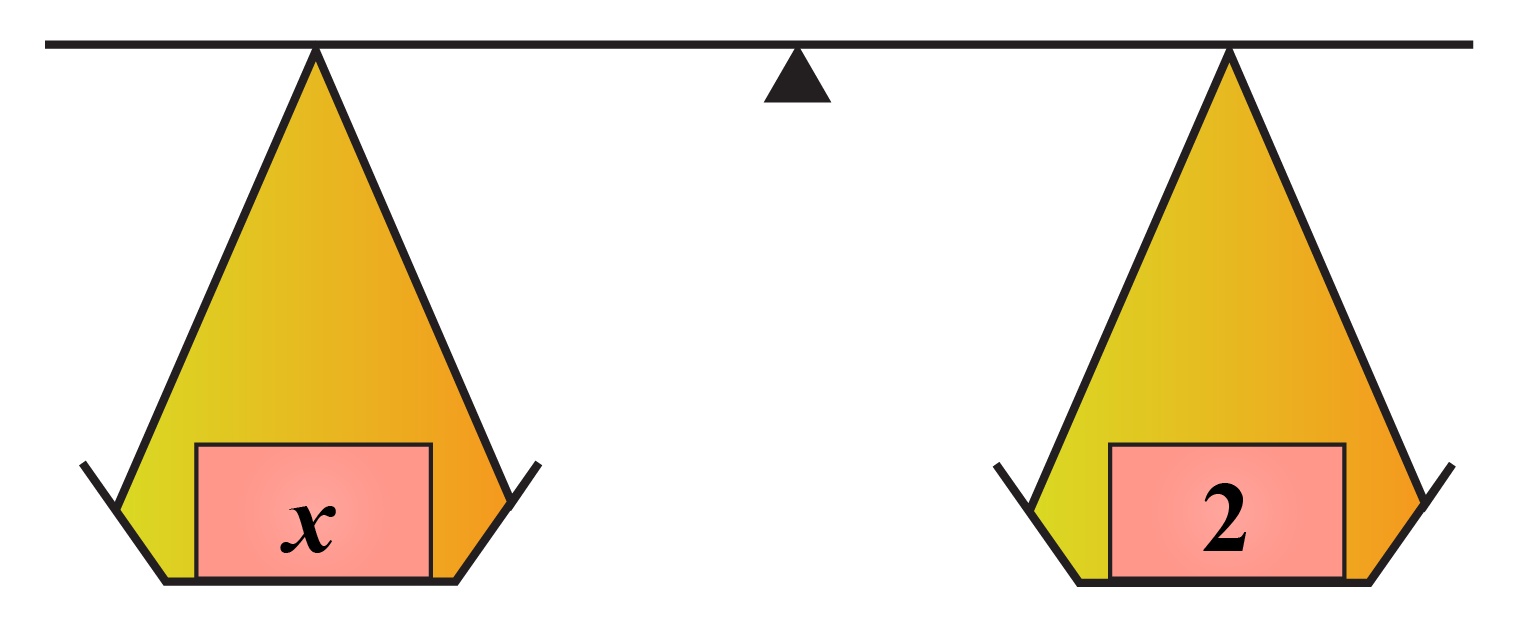Think Tank
1. Solve the following riddle.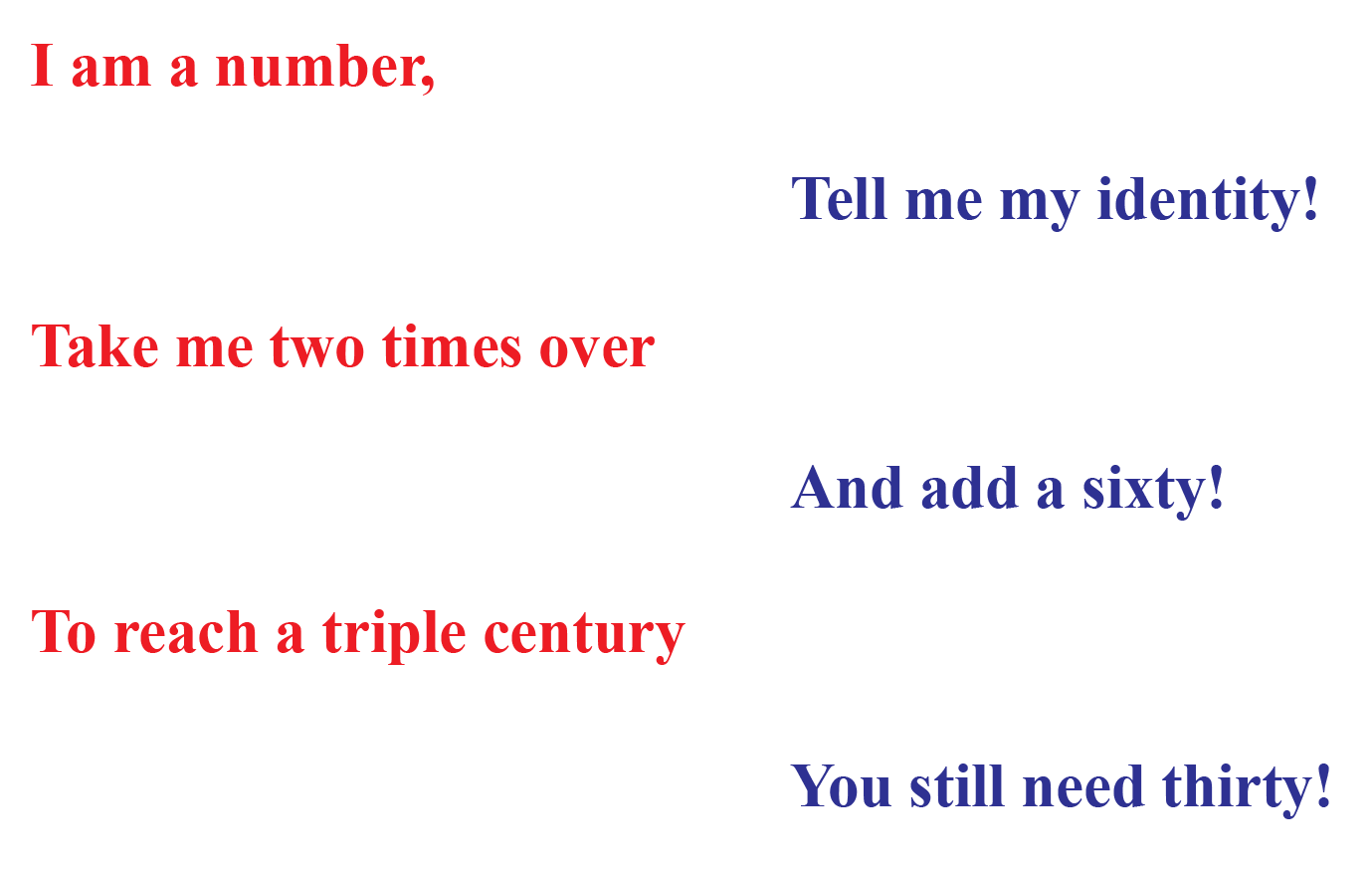## What Are the 3 Types of Equations?

There are 3 main types of equations in math.

### Linear Equation

The standard form of a linear equation with variables $$x$$ and $$y$$ is:

 $$Ax+By=C$$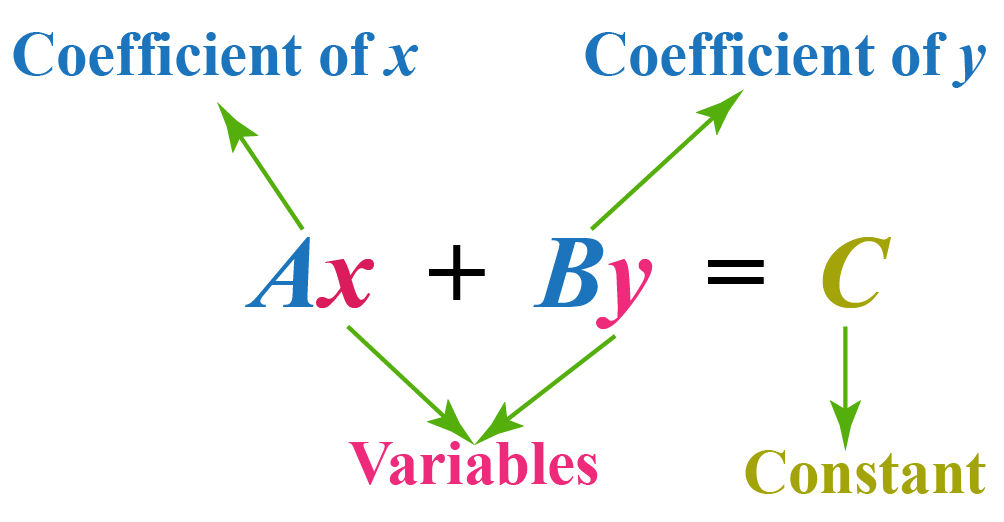The standard form of a quadratic equation with variable $$x$$ is:
 $$ax^2+bx+c=0$$, where $$a\neq 0$$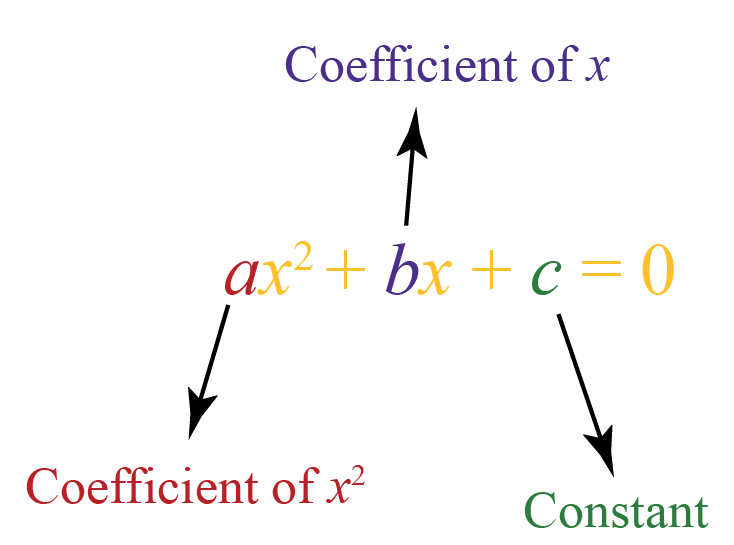### Cubic Equation

The standard form of a cubic equation with variable $$x$$ is:

 $$ax^3+bx^2+cx+d=0$$, where $$a\neq 0$$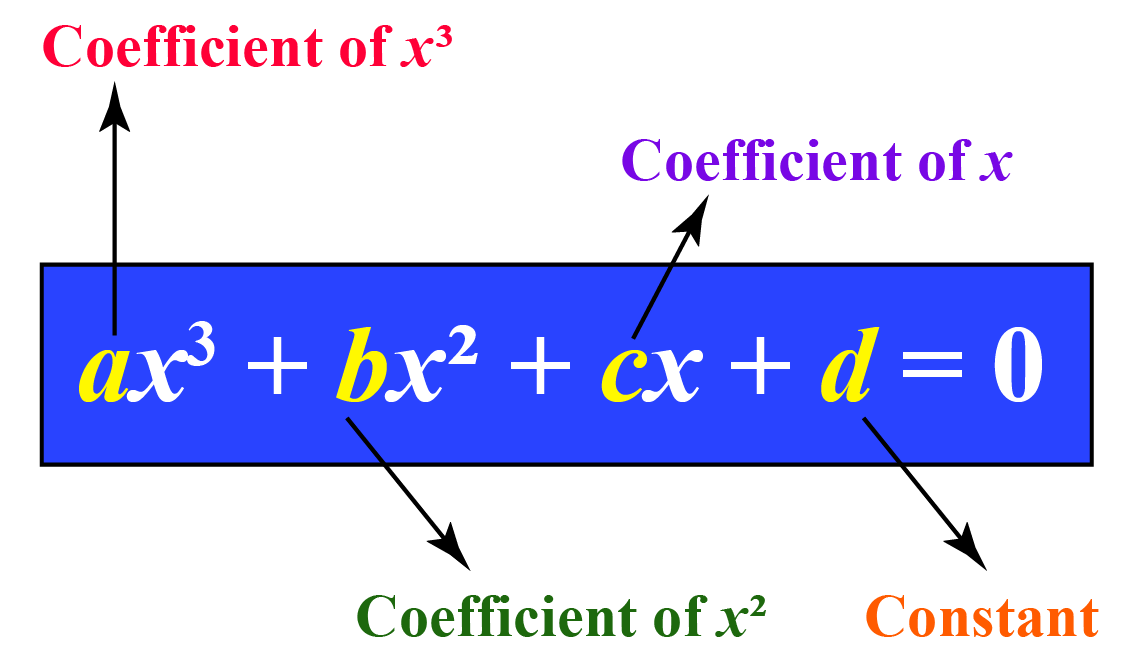More Important Topics
Numbers
Algebra
Geometry
Measurement
Money
Data
Trigonometry
Calculus
More Important Topics
Numbers
Algebra
Geometry
Measurement
Money
Data
Trigonometry
Calculus